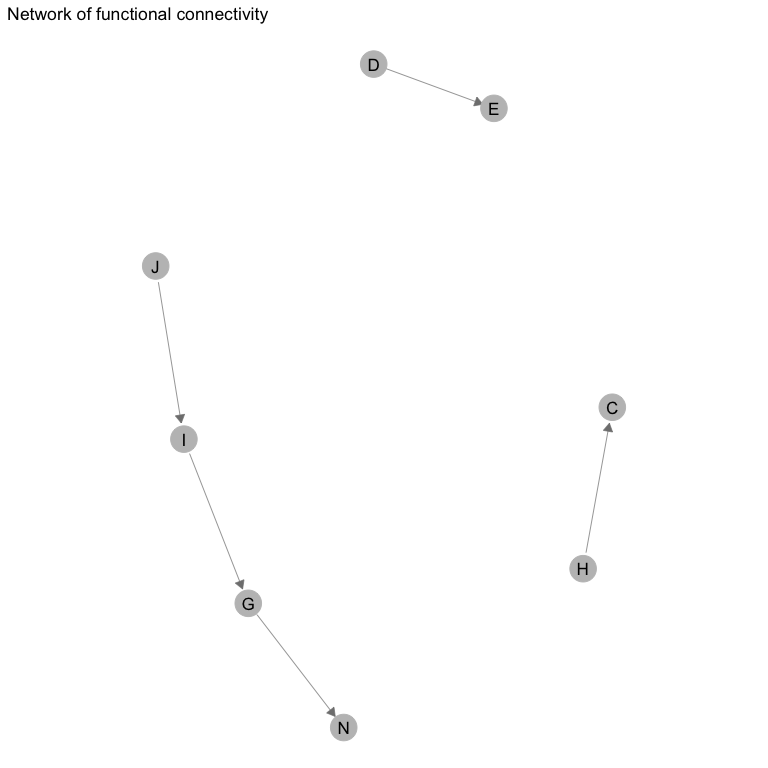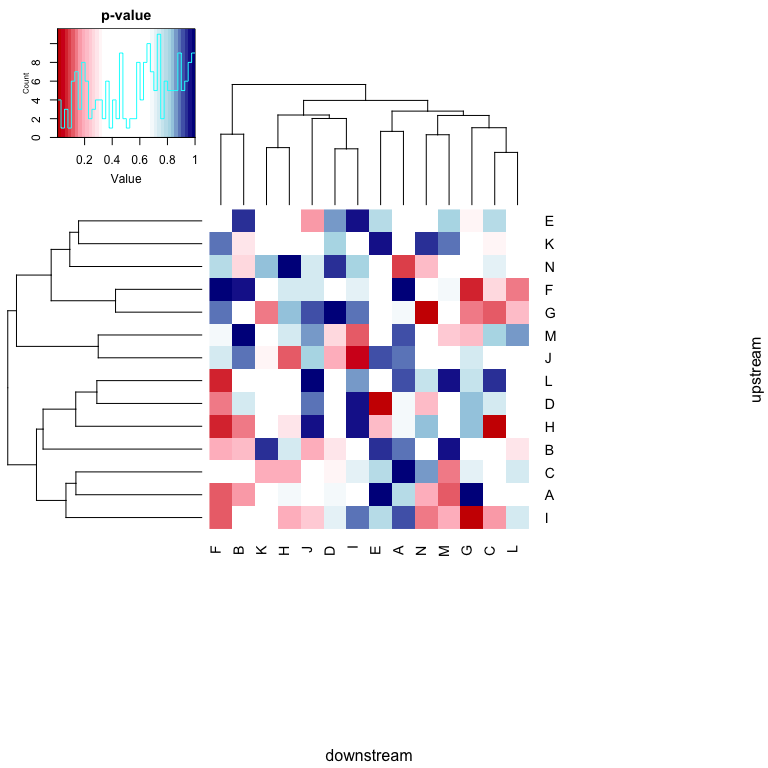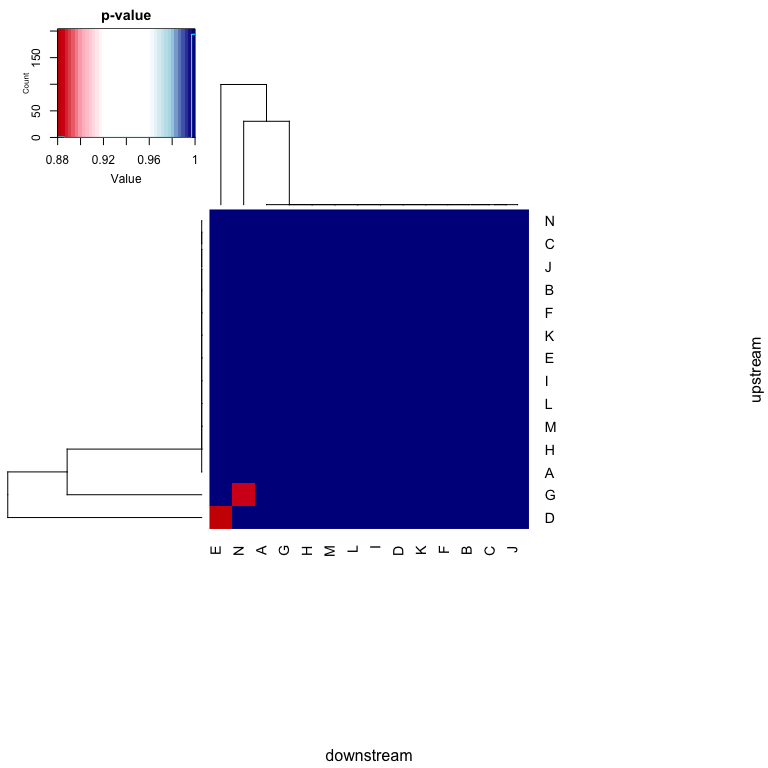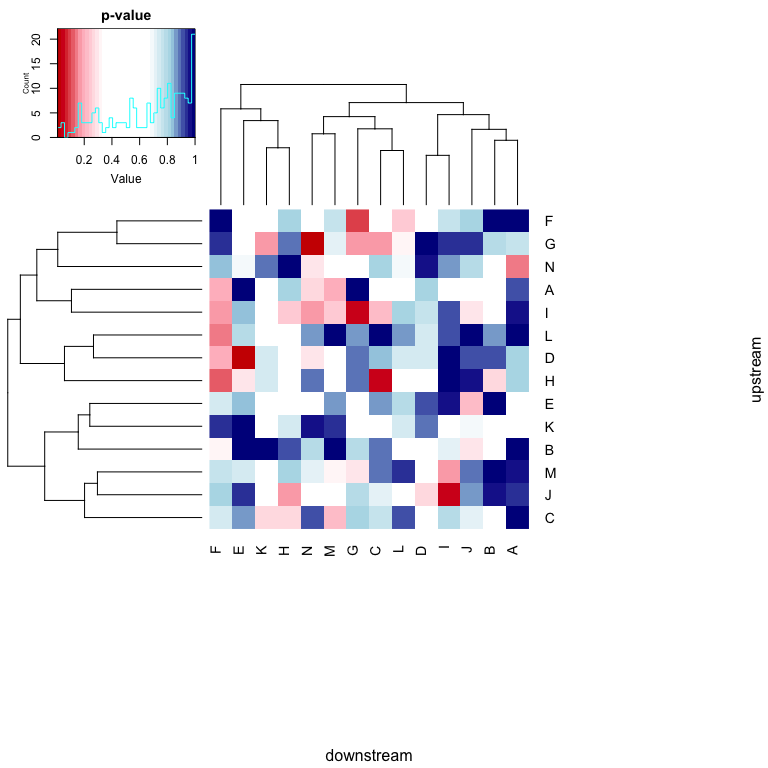# PAFway: Pairwise Associations Between Functional Annotations in Networks and Pathways

The purpose of this package is to allow the user to find pairs of annotations that are enriched in a network. For instance, the network might be a gene regulatory network, where the nodes represent genes and the edges represent regulatory interactions between pairs of genes. Each gene might have one or more functional annotation labels (such as GO terms) associated with them. The user might be interested in learning whether a specific GO term is enriched upstream of a second GO term. This function works with directed networks, with and without edge weights. The results can be depicted as either a network or a heatmap.

## Statistics explanation:

### No edge weights

When no edge weights are considered, enrichment between pairs of genes is determined by the binomial test.

### Edge weights

When a gene network contains edge weights, we calculate the sum of the edge weights of each edge type, and we would like to know whether this value is higher than would be expected by chance. For two functional annotations $$a$$ and $$b$$, let us define $$z_{a,b}$$ as the sum of the edge weights of edge type $$(a,b)$$ in the network. Let us say that $$c_{a,b}$$ is the count of the number of edges of that type. $$P(c_{a,b}=i)$$ is the probability of observing exactly $$i$$ edges of type $$(a,b)$$ and $$P(x \geq z_{a,b} | c_{a,b}=i)$$ is the probability of observing a sum of edge weights greater than $$z_{a.b}$$ given that $$c_{a,b}=i$$. The probability of observing at least $$z_{a,b}$$ is: % a weighted average of the probability of observing at least $$z_{a,b}$$ if there were exactly $$c_{a,b}$$ edges of edge type $$(a,b)$$ for all $$c_{a,b}$$ from $$1$$ to $$N$$ (where $$N$$ is the number of edges of the network). $\begin{equation} \label{eq:summation} P(x \geq z_{a,b})=\sum_{i=1}^N P(c_{a,b}=i) P(x \geq z_{a,b} | c_{a,b}=i) \end{equation}$

where $$N$$ is the number of edges in the network. Note that $$P(x \geq z_{a,b})$$ is the p-value.

## Example with a random network

First, let us construct a random network with 300 nodes, 1000 edges and 14 GO terms.

library(PAFway)

set.seed(123)
#Make 300 nodes
nodes=paste("node", c(1:300))
#First 3 node names:
print(nodes[1:3])
#>  "node 1" "node 2" "node 3"

#Assign them random GO terms
randomGO=c("A", "B", "C", "D", "E", "F", "G", "H", "I", "J", "K", "L", "M", "N")[sample(c(1:14), 300, replace=T)]
names(randomGO)=nodes
#First 3 nodes and associated GO terms:
print(randomGO[1:3])
#> node 1 node 2 node 3
#>    "C"    "N"    "C"

#Make 1000 edges
edgesRandom=t(sapply(c(1:1000), function(i){
nodes[sample(300, 2)]
}))
#First 3 edges:
print(edgesRandom[1:3,])
#>      [,1]       [,2]
#> [1,] "node 55"  "node 217"
#> [2,] "node 85"  "node 45"
#> [3,] "node 146" "node 170"

We can also consider a random network, where each gene can have more than one functional annotation:

#Assign each node a second GO term, separated by a '_' symbol
randomGO2=c("A", "B", "C", "D", "E", "F", "G", "H", "I", "J", "K", "L", "M", "N")[sample(c(1:14), 300, replace=T)]
randomGO_multiple=sapply(c(1:300), function(i){paste(randomGO[i], randomGO2[i], sep="_")})
names(randomGO_multiple)=nodes

#print first 5 elements, as a demo
print(randomGO_multiple[1:5])
#> node 1 node 2 node 3 node 4 node 5
#>  "C_B"  "N_H"  "C_J"  "J_A"  "B_J"

We can also select a sub-set of GO terms which we consider ‘interesting’:

#Interesting GO terms:
GO_interesting=c("B", "C", "D", "F")

PAFway works with and without edge weights. Here we generate random edge weights:

random_edge_weights=rnorm(length(edgesRandom[,1]), 1, 0.001)
print(random_edge_weights[1:5])
#>  0.9999511 0.9992959 1.0006808 1.0001300 1.0011097
print(length(random_edge_weights))
#>  1000

Now that we’ve set up some networks, we can see whether there are any enriched pairwise relationships between edges.

## Enrichment of pairwise associations without edge weights

First, we will use the main pafway function to find p-values for each function annotation pair. We will start off with the simplest example, which doesn’t use any optional parameters:

#This will run pafway, with no edge weights, for all the GO terms
a=pafway(randomGO, edgesRandom, unique(randomGO))
print(a[1:5, 1:5])
#>           C         N         J         B         F
#> C 0.6228151 0.8682946 0.6396453 0.3951836 0.6313025
#> N 0.7229578 0.2242200 0.7316659 0.2681023 0.7929657
#> J 0.6396453 0.4602521 0.8240874 0.8985991 0.7497069
#> B 0.6617244 0.6734212 0.1940617 0.2165586 0.1958142
#> F 0.2705119 0.4964210 0.7497069 0.9536428 0.9809709

This can be displayed as either a heatmap or a network:

draw_network(a)
#> Registered S3 method overwritten by 'GGally':
#>   method from
#>   +.gg   ggplot2draw_heatmap(a)
#> Warning in plot.window(...): "fig.height" is not a graphical parameter
#> Warning in plot.window(...): "fig.width" is not a graphical parameter
#> Warning in plot.xy(xy, type, ...): "fig.height" is not a graphical
#> parameter
#> Warning in plot.xy(xy, type, ...): "fig.width" is not a graphical parameter
#> Warning in title(...): "fig.height" is not a graphical parameter
#> Warning in title(...): "fig.width" is not a graphical parameterHowever, in this example, we do not correct for multiple hypothesis testing, so we get quite a few false positives, even though we know that we have started with a random network. Let us re-draw these, after adjustment for multiple hypotheses. We now see that no edges have a p-value<0.05.

draw_network(a, adjMethod = "bonferroni")
#>  "no edges are significant"
#> Warning in plot.window(...): "fig.height" is not a graphical parameter
#> Warning in plot.window(...): "fig.width" is not a graphical parameter
#> Warning in plot.xy(xy, type, ...): "fig.height" is not a graphical
#> parameter
#> Warning in plot.xy(xy, type, ...): "fig.width" is not a graphical parameter
#> Warning in title(...): "fig.height" is not a graphical parameter
#> Warning in title(...): "fig.width" is not a graphical parameter## Enrichment of pairwise associations with edge weights

Next, we’ll repeat the previous analysis, but with edge weights. We will start off with the simplest example, which doesn’t use any optional parameters:

#This will run pafway, with no edge weights, for all the GO terms
b=pafway_edge_weights(randomGO, cbind(edgesRandom, random_edge_weights), unique(randomGO))
print(b[1:5, 1:5])
#>           C         N         J         B         F
#> C 0.7650604 0.9190635 0.7208724 0.6295337 0.7313706
#> N 0.8016517 0.2812259 0.7861320 0.3916431 0.8460953
#> J 0.7208724 0.5286691 0.8637871 0.9588849 0.8056119
#> B 0.8802256 0.7966224 0.2890411 0.5452866 0.3266573
#> F 0.3658062 0.5742984 0.8056119 1.0000000 0.9917003

This can be displayed as either a heatmap or a network:

draw_network(b)draw_heatmap(b)
#> Warning in plot.window(...): "fig.height" is not a graphical parameter
#> Warning in plot.window(...): "fig.width" is not a graphical parameter
#> Warning in plot.xy(xy, type, ...): "fig.height" is not a graphical
#> parameter
#> Warning in plot.xy(xy, type, ...): "fig.width" is not a graphical parameter
#> Warning in title(...): "fig.height" is not a graphical parameter
#> Warning in title(...): "fig.width" is not a graphical parameterHowever, in this example, we do not correct for multiple hypothesis testing, so we get quite a few false positives, even though we know that we have started with a random network. Let us re-draw these, after adjustment for multiple hypotheses. We now see that no edges have a p-value<0.05.

draw_network(b, adjMethod = "bonferroni")
#>  "no edges are significant"
#>  "nothing is even close to being significant"

## More complex scenarios:

### More than one GO annotation per gene

If you use a larger database of GO terms, there may be more than one annotation per gene. These can be appended together, separated by a "_" symbol:

#Multiple GO terms are in: randomGO_multiple
b=pafway(randomGO_multiple, edgesRandom, unique(randomGO), exact=F)
print(b[1:5], b[1:5])
#>  1 1 1 1 0

### Only a subset of GO annotations are interesting

It is usually recommended that you only perform this analysis on GO terms that are of particular interest to you, because otherwise you will lose a lot of statistical power by comparing pairs of GO terms that you don’t care about.

#GO terms of interest are in GO_interesting
b=pafway(randomGO, edgesRandom, GO_interesting)
print(b[1:5], b[1:5])
#>  0 0 1 1 1

### Code is running too slowly (on a really big network)

If you are running pafway (specifically with edge weights) on a really big network, it can take a while. It might be worth decreasing accuracy and increasing speed. One way to do this is to change the threshold at which a value in a pdf is rounded to zero. Another way is to change the step size.

#This will run pafway, with no edge weights, for all the GO terms
b=pafway_edge_weights(randomGO, cbind(edgesRandom, random_edge_weights), unique(randomGO), step=0.001, thresholdZero=0.0001)
print(b[1:5, 1:5])
#>           C         N         J         B         F
#> C 0.7650604 0.9190635 0.7208724 0.6295337 0.7313706
#> N 0.8016517 0.2812259 0.7861320 0.3916431 0.8460953
#> J 0.7208724 0.5286691 0.8637871 0.9588849 0.8056119
#> B 0.8802256 0.7966224 0.2890411 0.5452866 0.3266573
#> F 0.3658062 0.5742984 0.8056119 1.0000000 0.9917003